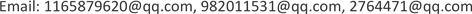1. 引言

2017年，美国波多黎各领土遭受飓风“玛利亚”重创，对岛上的建筑物、房屋和道路造成了大范围的破坏，电力服务中断，通信系统被摧毁，房屋倒塌，道路阻塞。数十个地区被孤立，没有沟通，而灾区又面临医疗用品、救生设备、能量供给品的需求。不仅仅是波多黎各，在面对自然灾害频发，地面救援无法执行的情况下，制定空中灾难救援应急系统就格外重要。

1) 设计一个DroneGo灾难响应系统，为每一个集装箱中设计相关的无人机装配方案。

2) 在波多黎各确定一个或多个最佳位置，用来放置三个集装箱。

3) 为无人机携带的药仓提供装箱计划并确定飞行计划。

2. 模型假设

1) 假设有无负载时飞行速度和时间保持不变

2) 假设无人机能按照预先给定路线飞行，不受其他外界因素干扰

3) 假设所有无人机的质量均相等，并且装箱存储时不会被压坏。

4) 假设无人机使用的货舱没有重量和厚度(存储在集装箱中的体积忽略不计)。

5) 假设货船沿海岸线均可停放集装箱。

3. 符号说明4. 模型的建立

4.1. 整数规划下的装箱模型 [<xref ref-type="bibr" rid="hanspub.31695-ref1">1</xref>]4.1.1. 约束条件与目标函数

Max U = α ( l i ω i h i β i ) V + ( 1 − α ) ∑ n i = 1 ( g i β i G )

∑ i = 1 n l i ω i h i β i ≤ V

a ′ ≤ ∑ i = 1 n g i x i β i ∑ i = 1 n g i β i ≤ a ″ ,   b ′ ≤ ∑ i = 1 n g i y i β i ∑ i = 1 n g i β i ≤ b ″ ,   ∑ i = 1 n g i z i β i ∑ i = 1 n g i β i ≤ c

4.1.2. 装箱模型的求解

1) 货物编号：将货物按体积由大到小降序排列， i = 1 , 2 , 3 , ⋯ , n

2) 设置方向编号：编号0代表货物不能旋转；编号1代表货物只能水平旋转；编号2代表货物能任意方向旋转。

Max U = α ( l i ω i h i β i ) V + ( 1 − α ) ∑ n i = 1 ( g i β i G )

4.2. TLBMCLP模型4.2.1. 模型的概述

Max ∑ i ∈ I ∑ j ∈ J h i d i j Y i j

∑ j ∈ J Y i j ≥ 1 , ∀ i ∈ I

∑ j ∈ J X j = p

Y i j − X j ≤ 0 , ∀ i ∈ I , j ∈ J

4.2.2. TLBMCLP模型的算法

Y i j = { 1 if     X i = 1     and     h i d i j − λ i > 0 0 else ∀ i ∈ I , j ∈ J

φ i = max { h i d i j } ,     ∀ i ∈ I

t k = α k ( Z U k − L B ) ∑ i ∈ I ( 1 − ∑ j ∈ J Y i j k ) 2 ， λ i k + 1 = max { 0 , λ i k − t k ( 1 − ∑ j ∈ J Y i j k ) }

{ ∑ j ∈ I Y i j k = 1 ,     ∀ i ∈ I U B − L B ≤ 0.3 t k ≤ 0.0001 k = 400

4.3. 无人机种类的选取

Drone performance tabl

A23.3350,6253.51
B52.6719,80081
C37.3390,000142
D18.0012,500111
E15.0013,500152
F31.6040,000222
G17.0717,408202
H0199,87500

Drone performance standard point

A−0.070.08−1.07
B1.770.56−0.48
C0.81−0.540.30
D−0.400.67−0.09
E−0.590.660.43
F0.450.241.35
G−0.460.591.09
H−1.52−2.26−1.53

4.4. 多目标规划模型

M a x ( min i ∈ I d h i )

d h i = min [ m k i r k i ]

p i j = f ( m k j , d i )

d i = min j { ( x h i − x c j ) 2 + ( y h i − y c j ) 2 }

N k j = ∑ j p i j χ k (i)

5. 模型的求解与分析5.1. 问题A的解决方案

MED distribution and demand tables for hospital

5.2. 问题B的解决方案

Maximum coverage point location and number of coverage point

Position of each containe

5.3. 问题C的解决方案5.3.1. 无人机有效载荷包装配置

Program for MED packagin

Puerto Rico儿童医院B：46\12\4692

E：13\7\
Puerto Rico儿童医院F：1511\\16588174
F：13\26
F：2\82

B：23\\2
Caribbean医疗中心F：95\449\48
F：22\6

5.3.2. 无人机飞行计划和时间表

Route schedul

237'52''
338'03''
Puerto Rico 儿童医院B124'34''
227'08''
329'47''

213'02''
313'25''
Puerto Rico 儿童医院F112'34''
213'47''
316'03''

231'54''
333'06''
Caribbean医疗中心F120'01''
222'17''
322'58''